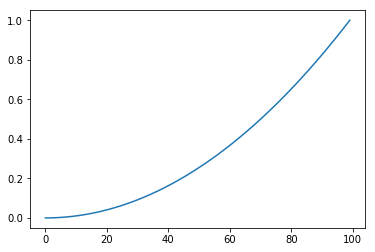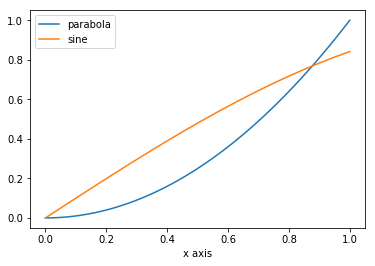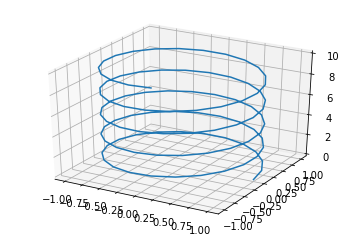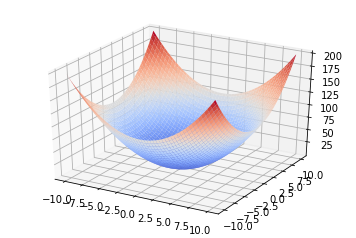In :
%matplotlib inline
import numpy as np;
from matplotlib import pyplot as plt


# CS 4540: Python Basics¶

Benjamin Bray, January 2017 for EECS 445 @ University of Michigan
(Updated August 2018 for CS 4540 @ Georgia Tech)

The goal of this tutorial is not to teach you everything you need to know about Python and the scientific libraries you will be using in this class, but rather to provide you with helpful resources and vocabulary you can use to search for help on your own.

## Installation¶

Even just a few years ago, installing Python was a nightmare, especially on Windows. Now, we are lucky enough to have Anaconda, a Python distribution that comes with many useful libraries and a package manager called conda that simplifies the installation of new libraries. For this class, we strongly recommend using Anaconda. Some warnings about Python:

• Python (like most software) works best on Linux :)
• Anaconda comes with a graphical interface for Python, but familiarizing yourself with the command line interface will make life much easier!
• On all platforms, installing Python may have unintended consequences on your platform PATH. This can be avoided by using conda environments or Python virtual environments, which also allow you to install different Python / library versions.
• This is useful, e.g. if you need to use Python 3 for one course and Python 2 for another, however...

Use Python 3! Unless you're running legacy code or need to use obscure, unmaintained libraries, there is absolutely no good reason to continue using Python 2, which will be officially retired in 2020.

For the rest of this tutorial, I will assume you are using Anaconda with Python >= 3.5.

## The Python Language¶

Python is an interpreted, dynamically typed, open-source language that is especially suitable for quick prototyping and scientific visualization. Python is very easy to read, which is why many people like to think of it as executable pseudocode.

"I came to Python not because I thought it was a better/acceptable/pragmatic Lisp, but because it was better pseudocode. Several students claimed that they had a hard time mapping from the pseudocode in my AI textbook to the Lisp code that Russell and I had online. So I looked for the language that was most like our pseudocode, and found that Python was the best match. Then I had to teach myself enough Python to implement the examples from the textbook. I found that Python was very nice for certain types of small problems, and had the libraries I needed to integrate with lots of other stuff, at Google and elsewhere on the net."Peter Norvig (emphasis added)

Python is not a functional programming language (like e.g. Haskell), but it does borrow some functional programming concepts like list comprehensions and lambda functions.

### Basic Language Features¶

Python has all the basic features you would expect it to have. In Python, there are no curly braces to indicate scope, and therefore whitespace matters. Here is some basic Python code:

In :
# recursively compute the nth Fibonacci number
def fibonacci(n):
if n <= 1:
return 1;
else:
return fibonacci(n-1) + fibonacci(n-2);

# print the first several Fibonacci numbers
for k in range(10):
print(k, fibonacci(k));

0 1
1 1
2 2
3 3
4 5
5 8
6 13
7 21
8 34
9 55


You should look up the following basic concepts in Python, only a handful of which I will demonstrate in this notebook:

In :
# list example
A = [1,2,3,4];
A

Out:
[1, 2, 3, 4]
In :
# list comprehension example
B = [ k**2 for k in range(10) ];
B

Out:
[0, 1, 4, 9, 16, 25, 36, 49, 64, 81]
In :
# dictionary example
"A" : 4.0,
"A-" : 3.7,
"B+" : 3.3,
"B"  : 3.0,
"B-" : 2.7,
"C+" : 2.3,
"C"  : 2.0,
"C-" : 1.7
}


Out:
4.0

### Python Semicolons¶

Semicolons are not strictly necessary in Python code, but I prefer to use them because my code feels "naked" otherwise. In interactive environments like IPython, Jupyter notebooks, or a terminal, the omission of a semicolon after a command will cause the return value of the command to be printed to the console. For example:

In :
5 + 7

Out:
12
In :
5 + 7;


### Fast Python¶

Python is remarkably fast for an interpreted language, but in general it cannot beat compiled languages designed with speed in mind. However, well-written Python code can come very close in speed to languages like C++ and Fortran. Python can also be extended directly with C/C++/Fortran code, using native Python C Extensions or using libraries like ctypes.

(you may enjoy the blog post Why Your Python Runs Slow, Part 1: Data Structures)

### Pythonic Code¶

You will often hear the phrase "Pythonic", referring to Python code that is particularly clear, and that conforms to the conventions established by the Python community. Pythonic code is not hard to write--if you are familiar with the language features, there is usually only one good way to perform a particular action. If you want to know what "Pythonic" code looks like, have a look at PEP 8: Style Guide for Python Code.

The following Python manifesto is built into the language itself:

In :
import this

The Zen of Python, by Tim Peters

Beautiful is better than ugly.
Explicit is better than implicit.
Simple is better than complex.
Complex is better than complicated.
Flat is better than nested.
Sparse is better than dense.
Special cases aren't special enough to break the rules.
Although practicality beats purity.
Errors should never pass silently.
Unless explicitly silenced.
In the face of ambiguity, refuse the temptation to guess.
There should be one-- and preferably only one --obvious way to do it.
Although that way may not be obvious at first unless you're Dutch.
Now is better than never.
Although never is often better than *right* now.
If the implementation is hard to explain, it's a bad idea.
If the implementation is easy to explain, it may be a good idea.
Namespaces are one honking great idea -- let's do more of those!


## Executing Python Code¶

There are several common ways to run Python:

• Interactively on the command line, using the Python REPL. Simply type python into a terminal.
• Some users prefer the IPython interactive terminal instead.
• Execute the contents of .py files all at once: python myscript.py
• Interactively in a Jupyter notebook, which is a mix of the above two approaches.

### Jupyter Notebooks¶

This document was written entirely inside of a Jupyter notebook (formerly known as IPython notebooks, but renamed because they now support many other languages)! These notebooks are great for documentation and prototyping because they allow you to mix Python code with math, text, and images! Use a mixture of Markdown, MathJax (which uses $\LaTeX$ notation), and HTML to mark up your notebooks.

$$\frac{\partial u}{\partial t} + (u \cdot \nabla) u - \nu \nabla^2 u = -\nabla w + g$$

If you aren't familiar with $\LaTeX$ math notation, you may find this cheat sheet helpful. You can also use Detexify to look up the commands by drawing symbols!

### Plotting with Jupyter¶

You can embed dynamically-created plots by calling the %matplotlib magic command at the top of a notebook (remember to call it before importing matplotlib, though!). We will cover matplotlib in more detail later.

%matplotlib from matplotlib import pyplot;

In :
plt.plot(np.linspace(0,1,100)**2)

Out:
[<matplotlib.lines.Line2D at 0x7fab43243ac8>]# Scientific Python¶

Python is used extensively in machine learning and the sciences for its scientific visualization capabilities. The following libraries will be very useful to you for EECS 445:

• The SciPy stack.
• NumPy for numerical linear algebra.
• Matplotlib for plotting and visualization, in particular the PyPlot interface.
• SymPy for symbolic math.
• Pandas for reading and processing data.
• Machine Learning
• SciKit-Learn contains implementations of almost every algorithm we will discuss in EECS 445.
• NLTK for natural language processing.

Many of these libraries come preinstalled with Anaconda, and the rest can be installed using the conda or pip package managers.

# Numpy Basics¶

The numpy library makes it easy to work with vectors and matrices. Many other libraries (like SciKit-Learn and pandas) make extensive use of numpy objects, so it is essential to familiarize yourself with its basic features. The conventional way to import numpy uses a shorter alias:

import numpy as np;

### numpy: Arrays¶

Numpy's basic data type is the ndarray ("n-dimensional array"), representing both vectors and matrices (and higher-dimensional objects like tensors). An ndarray is a homogeneous collection, in which all elements have the same data type and size.

### numpy: Manually-Defined Arrays¶

You can populate an array manually, just as you would with Matlab:

In :
A = np.array([ [1,2,3], [4,5,6], [7,8,9]]);
A

Out:
array([[1, 2, 3],
[4, 5, 6],
[7, 8, 9]])

### numpy: Data Types¶

If you do not provide a datatype, numpy will infer the data type for you. Be careful! Your calculations may not work as expected if numpy thinks you are working with integer arrays.

In :
A.dtype

Out:
dtype('int64')

Numpy allows you to explicitly define the type as follows:

In :
A = np.array([ [1,2,3], [4,5,6], [7,8,9] ], dtype=np.int64)
A.dtype

Out:
dtype('int64')

Alternatively, we could have used floating-point notation to define our array:

In :
B = np.array([1., 2., 3.]);
B.dtype

Out:
dtype('float64')

### numpy: Ranges¶

In :
# np.arange(start, stop, step) -- includes only the start value
np.arange(0,20,2)

Out:
array([ 0,  2,  4,  6,  8, 10, 12, 14, 16, 18])
In :
# np.linspace(start, stop, num) -- includes both endpoints
np.linspace(0,1,11)

Out:
array([ 0. ,  0.1,  0.2,  0.3,  0.4,  0.5,  0.6,  0.7,  0.8,  0.9,  1. ])

### numpy: Built-In / Special Matrices¶

In :
np.ones((3,3))

Out:
array([[ 1.,  1.,  1.],
[ 1.,  1.,  1.],
[ 1.,  1.,  1.]])
In :
np.zeros(10)

Out:
array([ 0.,  0.,  0.,  0.,  0.,  0.,  0.,  0.,  0.,  0.])
In :
np.eye(5)

Out:
array([[ 1.,  0.,  0.,  0.,  0.],
[ 0.,  1.,  0.,  0.,  0.],
[ 0.,  0.,  1.,  0.,  0.],
[ 0.,  0.,  0.,  1.,  0.],
[ 0.,  0.,  0.,  0.,  1.]])
In :
np.diag([1,2,3,4])

Out:
array([[1, 0, 0, 0],
[0, 2, 0, 0],
[0, 0, 3, 0],
[0, 0, 0, 4]])

### numpy: Random Matrices¶

In :
# random matrix with uniform[0,1] entries
np.random.rand(3,4)

Out:
array([[ 0.70554124,  0.86570049,  0.74322799,  0.24946316],
[ 0.9662329 ,  0.40675935,  0.69000641,  0.30783145],
[ 0.38888454,  0.80747942,  0.34995379,  0.50831056]])
In :
# random matrix with Gaussian-distributed entires
np.random.randn(4,2)

Out:
array([[ 0.55169944, -0.04391803],
[-0.60612155, -0.5541715 ],
[-0.36553767, -0.60184306],
[-0.6273487 , -1.40870078]])

### numpy: Matrix / Vector Operators¶

All of the standard operators are elementwise...

In :
np.diag(np.arange(3)) + np.array([ [1,2,3], [4,5,6], [7,8,9] ])

Out:
array([[ 1,  2,  3],
[ 4,  6,  6],
[ 7,  8, 11]])

...including multiplication.

In :
np.eye(3) * np.array([ [1,2,3], [4,5,6], [7,8,9] ])

Out:
array([[ 1.,  0.,  0.],
[ 0.,  5.,  0.],
[ 0.,  0.,  9.]])

To perform matrix-matrix and matrix-vector multiplication, use np.dot or the @ operator.

In :
A = np.random.randn(3,3);
x = np.random.randn(3);
np.dot(A, x)

Out:
array([-0.77608958,  3.25949369, -2.78771418])
In :
np.eye(3) @ np.array([ [1,2,3], [4,5,6], [7,8,9] ])

Out:
array([[ 1.,  2.,  3.],
[ 4.,  5.,  6.],
[ 7.,  8.,  9.]])

We can easily take the transpose of a matrix:

In :
A = np.random.randint(1,20,(3,5))
print(A);
print(A.T);

[[ 3 17 19 15  7]
[ 1 13  9 19 11]
[15  8  5 17  2]]
[[ 3  1 15]
[17 13  8]
[19  9  5]
[15 19 17]
[ 7 11  2]]


### numpy: Indexing and Slicing¶

Consider the following matrix:

In :
A = np.random.randint(-10, 10, (3,4))
print(A)

[[ 8 -9  3  9]
[ 2 -5 -8 -5]
[ 1 -2 -7 -1]]


We can easily obtain individual elements by indexing. Everything in Python, including numpy, is zero-indexed.

In :
A[1,2]

Out:
-8

We can obtain submatrices by slicing. For example,

In :
# second column
A[:,1]

Out:
array([-9, -5, -2])
In :
# third row
A[2,:]

Out:
array([ 1, -2, -7, -1])
In :
# submatrix (end index is not included)
A[0:2,1:3]

Out:
array([[-9,  3],
[-5, -8]])

### numpy: Slicing Weirdness¶

Be careful! Sometimes slices are just pointers to the original matrix, rather than copies.

In :
A = np.eye(3);
B = A[:,1];

print(A, B);

[[ 1.  0.  0.]
[ 0.  1.  0.]
[ 0.  0.  1.]] [ 0.  1.  0.]

In :
B = 100;
print(A, B);

[[   1.  100.    0.]
[   0.    1.    0.]
[   0.    0.    1.]] [ 100.    1.    0.]


To avoid this problem, you can explicitly copy ndarrays:

In :
A = np.eye(3);
A_copy = np.copy(A);
A_copy[0,1] = 100;

print(A);
print(A_copy);

[[ 1.  0.  0.]
[ 0.  1.  0.]
[ 0.  0.  1.]]
[[   1.  100.    0.]
[   0.    1.    0.]
[   0.    0.    1.]]


# Matplotlib Basics¶

The matplotlib library allows for the creation of basic graphs and charts in Python. The matplotlib API is almost an exact copy of Matlab's, so if you already know Matlab you should feel right at home!

I highly recommend reading the PyPlot tutorial.

### Basic Plotting¶

In :
x = np.linspace(0,1,100);
y1 = x ** 2;
y2 = np.sin(x);

plt.plot(x, y1, label="parabola");
plt.plot(x, y2, label="sine");
plt.legend();
plt.xlabel("x axis");### 3d Plotting¶

In :
# import 3d stuff
import matplotlib.pyplot as plt
from mpl_toolkits.mplot3d import Axes3D

# create figure; enable 3d
fig = plt.figure()

# plot parametric curve
t = np.linspace(0, 10, 100);
x = np.cos(t * 3);
y = np.sin(t * 3);

ax.plot(x,y,t)

Out:
[<mpl_toolkits.mplot3d.art3d.Line3D at 0x7fab430b3470>]### Plotting Surfaces¶

In :
# need to define a grid on the xy plane
xvals = np.linspace(-10,10, 100);
yvals = np.linspace(-10,10, 100);

X,Y = np.meshgrid(xvals, yvals);
Z = X**2 + Y**2;

# enable 3d
fig = plt.figure()
ax.plot_surface(X, Y, Z, cmap="coolwarm");# Practice¶

Create a 5x5 matrix with 1,2,3,4 just below the diagonal.

In :
np.diag([1,2,3,4], k=-1)

Out:
array([[0, 0, 0, 0, 0],
[1, 0, 0, 0, 0],
[0, 2, 0, 0, 0],
[0, 0, 3, 0, 0],
[0, 0, 0, 4, 0]])

Create an 8x8 matrix and fill it with a checkerboard pattern.

In :
A = np.zeros((8,8))
A[::2,::2] = 1
A[1::2,1::2] = 1
A

Out:
array([[ 1.,  0.,  1.,  0.,  1.,  0.,  1.,  0.],
[ 0.,  1.,  0.,  1.,  0.,  1.,  0.,  1.],
[ 1.,  0.,  1.,  0.,  1.,  0.,  1.,  0.],
[ 0.,  1.,  0.,  1.,  0.,  1.,  0.,  1.],
[ 1.,  0.,  1.,  0.,  1.,  0.,  1.,  0.],
[ 0.,  1.,  0.,  1.,  0.,  1.,  0.,  1.],
[ 1.,  0.,  1.,  0.,  1.,  0.,  1.,  0.],
[ 0.,  1.,  0.,  1.,  0.,  1.,  0.,  1.]])

Write a function to generate a random orthogonal matrix of a specified size.

In :
def random_orthogonal(n):
A = np.random.randn(n,n);
Q,R = np.linalg.qr(A);
return Q;